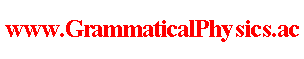since 2006 Help　Sitemap < Forum > < Definition > < Abstracts for the Meetings Held by the Physical Society > < JPS 2006 Spring Meeting > 27pXA-6 New Quantum Grammar Uda's Special School / Yuichi Uda --- As a practice of grammatism, this time, I propose an improvement in the grammar of quantum mechanics. This improvement is essentially applicable not only to quantum mechanics but to all quantum theories including quantum field theory. As everybody knows, a quantum state of a system with n degrees of freedom is represented by a wave-function that is a mapping from R^n to C. Let Ψ represent this wave-function, and quantum state Ψ is represented by the following proposition: "The quantum state of the first degree of freedom is ψ(□,1) and the quantum state of the second degree of freedom is ψ(□,2) and ・・・ and the quantum state of the n-th degree of freedom is ψ(□,n)." that is ∀j; "The quantum state of the j-th degree of freedom is ψ(□,j)." if Ψ can be written as follows. Ψ(x(1),x(2),・・・,x(n))=ψ(x(1),1)ψ(x(2),2)・・・ψ(x(n),n) I express such situations by the sentence "quantum state Ψ is analyzable." And a quantum state is not always analyzable generally. There is nothing new until here. Now, imitating above, I assume that a functional which maps each mapping from R to R onto a complex number represents a quantum history of a system with one degree of freedom. This is the new grammar which I propose this time. As a feeling, if Φ denotes this functional, and if Φ can be written as Φ[x] ＝ Π φ(x(t),t), 　　　　　 t this quantum history Φ is represented by the following proposition. ∀t; "Quantum state at time t is φ(□,t)." To mean this situation, I say "Quantum history Φ is analyzable." And I propose an assumption that a general quantum history, too, is not always analyzable. However, appearance of infinite product does not seem real not in an expression as a feeling but in a precise expression. So, I assume accurately that if quantum history Φ is written as Φ[x] ＝ exp[α∫dt φ'(x(t),t)], Φ is analyzable and Φ corresponds to φ such that ∀ξ,t∈R; φ(ξ,t) ＝ exp φ'(ξ,t). Here, φ is a representation of the quantum history in the old grammar. α is a new fundamental constant of physics, and I named it the analysis constant. --- Shift JIS is the suitable encoding for viewing this message. --- This article is a rewrite of the following article. JPS 2006 Spring Meeting@Abstracts for the Meetings Held by the Physical Society@Definition of Grammatical Physics@Grammatical Physics@Forum@Vintage(2008-2014) Author Yuichi Uda, Write start at 2015/05/25/18:36JST, Last edit at 2015/05/25/18:36JST